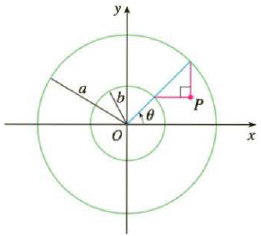Chapter 10.1, Problem 41E

Chapter
Section
Textbook Problem

If a and b are fixed numbers, find parametric equations for the curve that consists of all possible positions of the point P in the figure, using the angle θ as the parameter. Then eliminate the parameter and identify the curve.To determine

To find: The parametric equation of the cycloid for the variables x and y and eliminate the parameter and identify the curve.

Explanation

Calculation:

Consider the geometry of the circle shown below in Figure 1.

From Figure 1, radius of the inner circle |OS| is b and radius of the outer circle |OR| is a and length of the segment, |RS|=ab.

In triangle SOT,

Length of the bottom side,

|OT|=acosθx=αcosθ (1)

Length of the vertical side, |ST|=asinθ

In triangle SRP,

Length of the bottom side,|SP|=(absinθ)

Length of the diagonal,

|PT|=STSP=asinθ(ab)sinθ=asinθasinθ+bsinθy=bsinθ (2)

The co-ordinates of point P (αcosθ,bsinθ)

From equations (1) and (2), The parametric equation for th

Still sussing out bartleby?

Check out a sample textbook solution.

See a sample solution

The Solution to Your Study Problems

Bartleby provides explanations to thousands of textbook problems written by our experts, many with advanced degrees!

Get Started

Find the general indefinite integral. (x2+1+1x2+1)dx

Single Variable Calculus: Early Transcendentals, Volume I

Sometimes, Always, or Never: If an ≥ bn ≥ 0 for all n and {bn} diverges, then {an} diverges.

Study Guide for Stewart's Single Variable Calculus: Early Transcendentals, 8th

Using a binomial series, the Maclaurin series for is:

Study Guide for Stewart's Multivariable Calculus, 8th